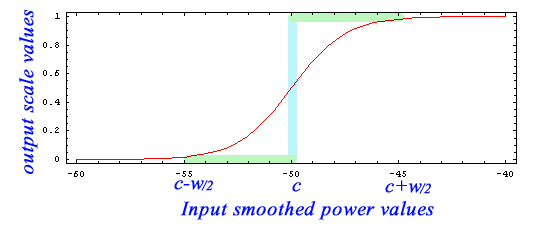MzPowerCurve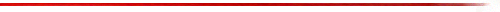### SYNOPSIS

MzPowerCurve -- Measures the power over time of an audio signal.
Generates function data for various curves derived from the power in the audio signal.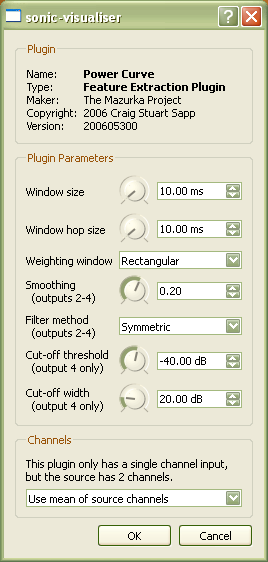### INPUT PARAMETERS

MzPowerCurve accepts 7 input parameters:

1. Window size
The size of the analysis window in milliseconds over which the power will be calculated.
2. Window hop size
The step size between analysis windows starting points in milliseconds.
3. Weighting window
Selects the analysis window which will be applied to the data before the power measurement is taken. A "Rectangular" window does not scale the audio data, and is used for non-weighted power measurements.
4. Smoothing
Used in outputs #2-4. Feed-forward gain for the filter used to smooth the raw power curve.
5. Filter method
Used in outputs #2-4. Specifies if the smoothing should be done in the forward direction, reverse direction, or symmetric (first reverse, then forward).
6. Cut-off threshold
Used only for output #4 (scaled smoothed power slope). This is the noise floor level in dB. Slope data below this cut-off level will be scaled towards 0 to minimize slope peaks caused by noise.
7. Cut-off width
Used only for output #4 (scaled smoothed power slope). The is the transition width at the cut-off threshold level for scaling the slope data. If the signal is louder than the threshold plus 1/2 of the cut-off width, then the slope data will not be altered. If the slope data is less than the threshold minus 1/2 of the cut-off width, then the slope data is scaled to 0. Slope data around the threshold transitions between 0 and 1 according to the cut-off width parameter.

### OUTPUTS

MzPowerCurve generates 4 outputs:

1. Raw power
A function of the average raw power for each input signal block.
2. Smoothed power
A time-symmetric exponentially smoothed function of the average raw power (output #1) for each input signal block.
3. Smoothed power slope
The slope of the smoothed power curve (output #2). Peaks in the slope indicate regions of sudden increases in loudness (such as note attacks).
4. Scaled power slope
The scaled slope of the smoothed power curve (output #3). Similar to output #3, but slope peaks in low amplitude signals are suppressed.

### DESCRIPTION

The equation for average power in decibels for a region of audio data is: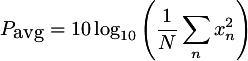where N is the number audio samples to average over, and xn are the individual audio samples. The term inside the log function has several alias meanings: average (plain English), mean (mathematics, statistics), arithmetic mean (mathematics), and sample mean (statistics). The average power is converted to decibels in the plugin, since hearing loudness (related to power) occurs on a logarithmic scale.

In addition to average power, the MzPowerCurve plugin can also calculate weighted average power: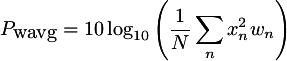where wn is the n-th value of a weighting function. The Weighted average power can be used to give a smoother measurement of the power at a particular point. Here is a schematic showing the difference between the average power and the weighted average power: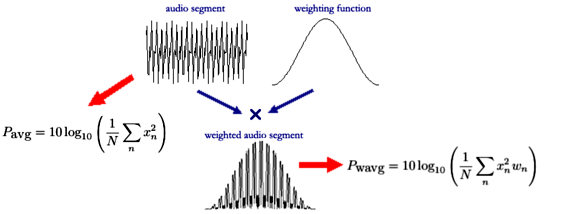1. Raw power curve

The first output feature of the MzPowerCurve plugin is the raw power. The power is measured by chopping the signal up into little pieces and measuring the average power in each of those pieces. No further processing of the power measurement is done, hence the name raw.

The timestamp for the individual raw power measurements is set to the middle of the region (block) of audio signal over which the average power was measured as shown in the following schematic of the extraction of raw power from the signal: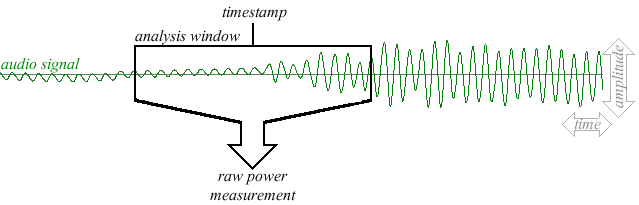As a technical programming aside, note that the vamp plugin system sends the process() function a timestamp of the start of the analysis window, so one-half of the duration of the window has to be added to that time so that it is centered in the middle of the window instead.

The analysis windows used to calculate each raw power measurement are separated by the step size between windows -- the number of samples by which the analysis window is shifted through the audio signal for each measurement. If the step size is less than the block size (analysis window length), then the analysis windows are said to overlap as shown in the following figure. If the step size is equal to the block size, then there is 0% overlap between the analysis windows.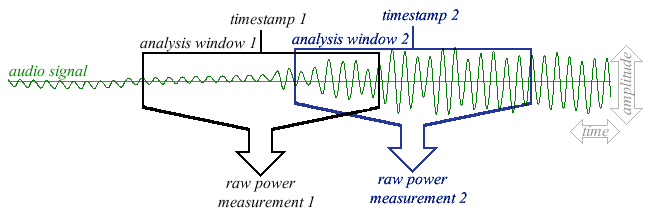The following figure display from Sonic Visualiser shows the raw power curve for a sinusoid with a triangular linear amplitude envelope. The cursor is pointing to the power level at the peak amplitude which is at about 3 dB. The audio waveform is shown in green, while the power curve is displayed in orange. The mouse is pointing to the peak amplitude, where the raw power was measured to be -2.95784 decibels (see numbers in top right corner).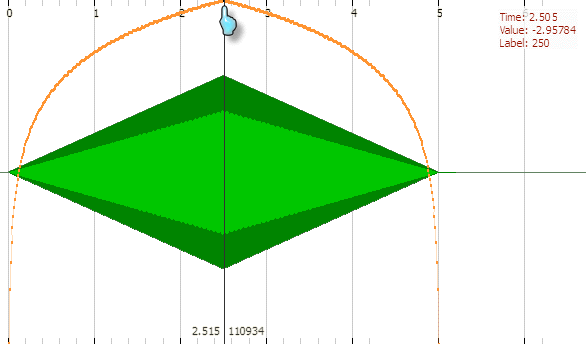In real audio, you will have to choose a block size which is small enough to be reactive to what is actually happening in the signal. For example, taking the average power of an entire composition is not an extremely useful exercise. You want to have a smaller analysis window so that changes in amplitudes caused by things such as note attacks are visible in the curve. In general 10-50 milliseconds is a good range for the duration of the analysis window. The step size is not as important, but small step sizes will give you a clearer picture of the change in the power curve over time.

Here is a figure demonstrating a smallish window size which is sensitive to beating in the audio signal. The orange line is the raw power measurements (actual measured values are located at the points). If you want to see the effects of beating you need a window around 1 to 20 Hz, depending on the rate of beating that you are interested in. To avoid looking at beating effects, you should have a window size of no less than 10 milliseconds.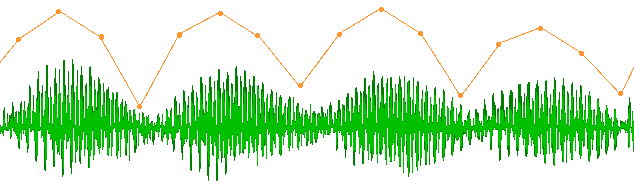2. Smoothed power curve

The raw power is, in fact, raw. In real audio data, the raw power curve can jump around a lot due several factors such as beating and noise. Therefore, it is usually useful to smooth out the raw power data to get a view of the power curve which is more perceptually relevant. This can be done by increasing the window size which will decrease the reactivity of the curve, or in the case of MzPowerCurve's output #2, the raw power curve is low-pass filtered with an exponential smoothing filter. The following figure demonstrates the difference between the raw power measurement (black curve) and the smoothed power data (orange curve, dots being the actual values).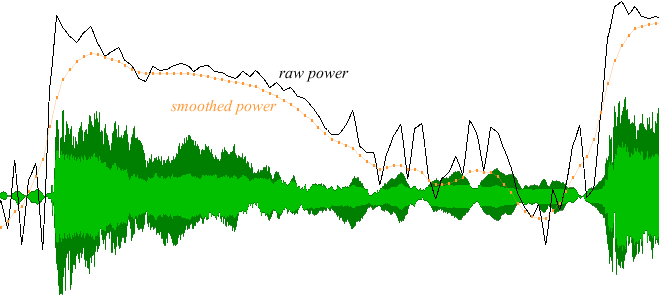The above figure shows the complete attack and decay of a single chord plus the beginning of the attack of the following chord played on a piano. Notice that the raw power curve becomes jagged as the note decays. This jaggedness is usually not interesting to see, so the smoothed curve mostly ignores behavior like that. Also note that the vertical scales of the black and orange curves are not exactly the same, so ignore vertical differences between the curves.

The exponential smoothing filter used in MzPowerCurve is described by the following difference equation: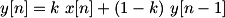where x[n] is the current filter input, y[n] is the current filter output, y[n-1] is the previous filter output, and k is a scaling factor set to 0.3 in the basic power curve case. This filter is applied twice to the power data: both forwards and backwards so as to remove any filter delay effects. If you do not do equivalent forward/reverse filtering on the data, various frequencies components of the smoothed power curve will be delayed by different amounts. The exact delay characteristics of the filter can be calculated from the difference equation given above. Here are the delay characteristics when using a step size of 10 ms, and a filter gain value of k = 0.3: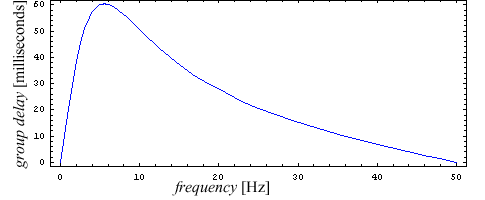What the above plot shows is if the raw power values remain constant (at 0 Hz), there will be no delay in the smoothed version of the curve. However, if the raw power samples are oscillating at a rate around 8 Hz, then the smoothed version of the curve will delay these values by around 60 milliseconds. So if you need to know when something occurs in the audio very accurately, you do not want the time smearing caused by the smoothing filter. This frequency dependent time-delay is removed by applying the same filter in reverse on the data. In other words, the forward filtering will delay 8 Hz oscillations by 60 milliseconds, but the reverse filtering will delay 8 Hz oscillations by -60 milliseconds. Therefore the net movement of the smoothed curve becomes 0 for all frequency components.

Actually, any low-pass filter could be used, not just the exponential smoothing filter shown above. All you have to do is apply 1/2 of the filtering in the forward direction and 1/2 of the filtering in the reverse direction. Feed-back filters where the current output is dependent on a previous output, such as the case for the exponential smoothing filter, must be processed in this way to remove the delay effects of the filter. The delay of all frequencies in a feed-forward filter (such as averaging: y[n] = (x[n] + x[n-1])/2 ) is constant, so you can just subtract a single time constant to properly align the smoothed curve with the raw curve without doing reverse filtering.

A useful application of the smoothed power curve is to examine the decay rates of notes in a piano recording. For example the following figure shows two different decay rates on two successive notes. The black curve is the smoothed slope in this case. The orange lines indicate note attacks, and the red lines highlight the two different decay slopes. In this case the first note is played staccato, and the second note is played sustained.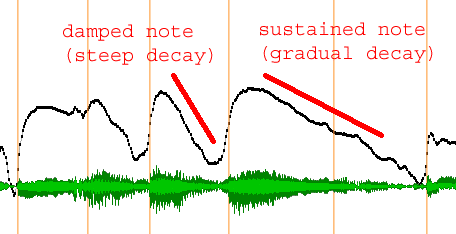3. Smoothed power slope curve

The third feature output from MzPowerCurve is a measurement of the slope of the smoothed power curve data. The slope measurement is used to help identify note attacks in the audio signal. Examine the following figure which displays the raw and smoothed power curves. From which curve is it easier to pick out the note attack location?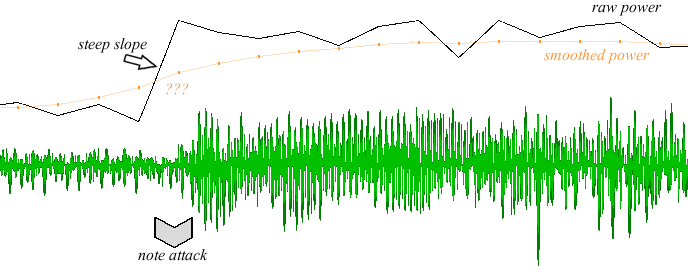It may look like the raw power curve is the best one to use to localize the location of the note attack because the slope is steep and the jump is large. The orange curve seems apparently useless in this situation because the slope is barely rising, and the seems to pass through the attack region hardly being affected by it. The orange curve also seems to have smeared the sharp attack seen in the raw power curve, with the smearing occurring both before and after the attack onset. A problem with using the raw power curve is that the raw power can jump up and down for no apparent reason, giving lots of false positives if you are trying to automatically find note attacks.

The sneaky thing that has to be done is to examine the slope of the smoothed power curve. You do not want to look at the absolute positions in the smoothed curve, but rather the inflection points which turn into peaks in the plot of the slope. The smoothed curve did smear the attacks, but it smeared them in both directions in exactly equal amounts of smearing. Looking at the slope of the smoothed curve will clearly show you where the midpoint of the smeared attack is located. The following figure displays, in purple, the slope of the orange curve (on a different vertical scale). Notice that there is a sharp peak in the smoothed power slope curve in the region of the note attack.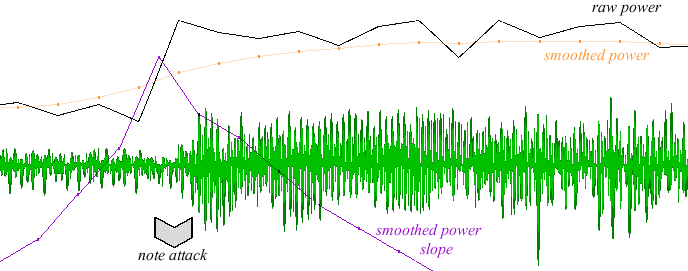Note that if you do not do the time symmetric smoothing as described in the above section, the peaks in the slope curve will (1) be delayed from the actual onset, and (2) will be smudged due to the frequency independent time delay of each frequency in the power-curve signal. (For feed-forward low-pass filters, there would be no smudging).

Below is an example attack region in an audio file with the three types of filtering that MzPowerCurve can do. Note that in this case the filter parameter was set to 0.2 for the symmetric filtering, and the filter parameter was set to 0.1 for the forward and reverse filtering to make them comparable to the symmetric filtering which does the filtering twice (once in each direction).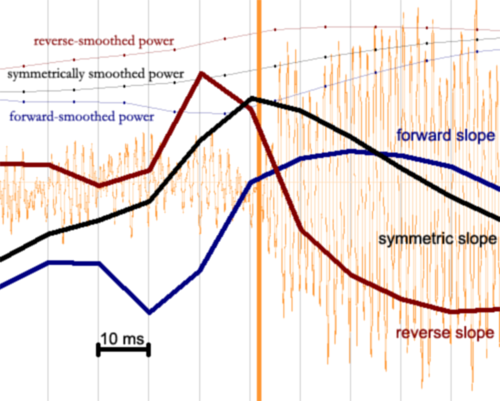Looking at the smoothed power slope is not a panacea for identifying note attacks. It is best used on percussive instruments such as the piano, and it does not work well in regions of dense note attacks since the slope peaks might blur together. However, it is excellent at localizing notes in slow, quiet contents. The following figure demonstrates this property where a quiet note follows a louder note at a quarter note duration. The second note is not visible in the raw power curve (black) or the smoothed power curve (orange), but in the smoothed power slope curve (purple) there is a sharp peak identifying the attack onset.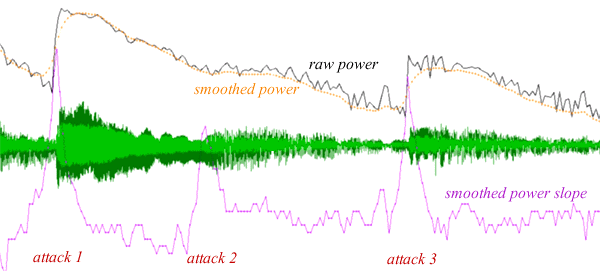The slope curve is somewhat insensitive to the effects of beating and resonances. In the following figure you can see the sharp attack peak at the beginning of a note. There are three or so resonance (or beating) peaks where the sound sustains better than normal, and the amplitude of the note actually increases seemingly defying the laws of physics. In the purple slope curve, these resonances are not as sharp as a regular attack, and the slope is also close to zero in this example.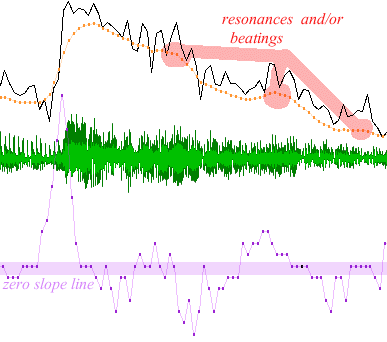Another application of the smoothed power slope is for identification of non-simultaneous events in the performance -- particularly the left-right hand coördination of chords. The following example shows two beats. The first beat contains notes for both the left and right hand. For this beat, the pianist plays the right hand note about 70 milliseconds before the left hand notes (each dot on the curve represents 10 milliseconds). Also, the left hand notes appear to be spread out about 30 milliseconds or so, but the resolution of the curve is not sufficient to resolve time differences that small with the given analysis settings. Note that the single note played on the next beat has a sharp single peak.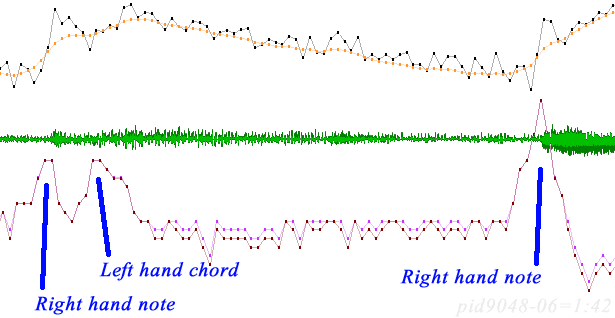4. Scaled power slope curve

The fourth output of the MzPowerCurve plugin is nearly identical to the slope of the smoothed power curve which is output #3, but the slope has been scaled by the smoothed power data. The following figure demonstrates the four outputs from MzPowerCurve. Notice that in the region of silence to the right in the figure, the scaled power slope is constant (at 0), while the other curves are jumping around due to being near the noise floor.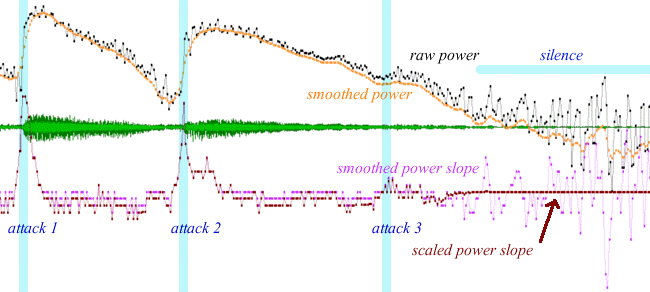Notice that the scaled power slope does not have peaks when the amplitude of the signal is low. For example, in the region after attack #3 in the figure above, the smoothed power slope has several peaks in the silent region. These are spurious peaks due only to the noise present in the silent regions. The scaled power slope is designed to remove these spurious peaks while preserving peaks which are present in louder sections of the audio.

The scaled power slope is created by multiplying the smoothed power slope by a form of the smoothed power. The smooth power range is scaled to the range between 0 and 1 using the sigmoid function: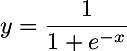Two parameter controls are added to the sigmoid function to control is effect on the scaled power slope: (1) a value c which is the cutoff threshold between silence/non-silence, and (2) a value w which is the threshold window sensitivity factor around the cutoff threshold; the transition width between the scaled values of 0 and 1.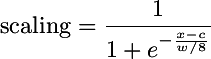For example, if c = -50 dB, and w = 10 dB, the sigmoid would be centered at -50 dB, and have a transition region of 10 dB. The Scaling values for power below -55 dB would be close to zero, and values above -45 dB would be close to 1, with the range from -55 to -45 dB being the transition region between silence/non-silence as illustrated in the following plot: